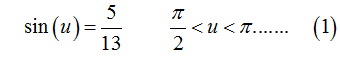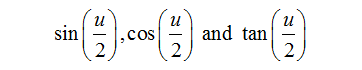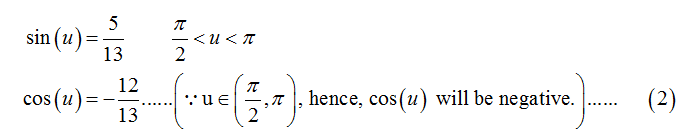# Use the given conditions.sin(u) =5/13, pi/2< u < π(b) Find the exact values of sin(u/2), cos(u/2), and tan(u/2) using the half-angle formulas.

Question
79 views

Use the given conditions.

sin(u) =5/13, pi/2< u < π
(b) Find the exact values of
sin(u/2), cos(u/2), and tan(u/2) using the half-angle formulas.
check_circle

Step 1

The given trigonometric value isStep 2

To find the value of following using half-angle formulas.Step 3

Since “u” lies in 1st quadrant, therefore sine trigonometric function will give positive value, while cosine and tangent trigonometric function will give a negative value.

Therefore, from (1)...

### Want to see the full answer?

See Solution

#### Want to see this answer and more?

Solutions are written by subject experts who are available 24/7. Questions are typically answered within 1 hour.*

See Solution
*Response times may vary by subject and question.
Tagged in